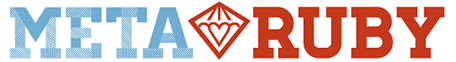# Need help understanding recursian

Hello,
I have trouble understanding the following code for 2 reasons:
I don’t exactly understand the task nor do I understand the way the algorithm operates.
I would really appreciate if somebody could break it down for me.
Thanks

``````# Catsylvanian money is a strange thing: they have a coin for every
# denomination (including zero!). A wonky change machine in
# Catsylvania takes any coin of value N and returns 3 new coins,
# valued at N/2, N/3 and N/4 (rounding down).
#
# Write a method `wonky_coins(n)` that returns the number of coins you
# are left with if you take all non-zero coins and keep feeding them
# back into the machine until you are left with only zero-value coins.
#
# Difficulty: 3/5

def wonky_coins(n)
return 1 if n == 0

# call wonky_coins from inside itself. This is called *recursion*.
return wonky_coins(n / 2) + wonky_coins(n / 3) + wonky_coins(n / 4)
end

puts("\nTests for #wonky_coins")
puts("===============================================")
puts "wonky_coins(1) == 3: "  + (wonky_coins(1) == 3).to_s
puts "wonky_coins(5) == 11: "  + (wonky_coins(5) == 11).to_s
puts "wonky_coins(6) == 15: "  + (wonky_coins(6) == 15).to_s
puts "wonky_coins(0) == 1: "  + (wonky_coins(0) == 1).to_s
puts("===============================================")``````

First off, just to get the record straight, it’s called recursion.

Recursion, in programming, is the act of calling one self with a smaller problem.
One way of showing this, is with the Fibonacci sequence.

We know that the next number in the Fibonacci sequence is the sum of the previous two.
We start with `1, 1` and now need to figure out what the next term is. It should be the sum of the two previous, which is `1+1=2`, so the next term is `2`. The next terms again is then `1+2=3`, `2+3=5`, `3+5=8`, and so on.
We can write this as a recursive function. A recursive function usually have a base case. Here the base case is that the first (0th) and second (1st) term are `1`.

Lets write this is Ruby:

``````def fib(n)
return 1 if n == 0
return 1 if n == 1
# do something more ...
end
``````

What should the “do something more” be? Well, it should take the two previous terms and add them. How do we get those? By calling `fib`, but with smaller numbers (smaller problem). If `n=2`, we should have `fib(2) = 2` as well. The two previous terms are `n=0` and `n=1`, so we could write `fib(2) = fib(0) + fib(1)`. To do this generally, we could say `fib(n) = fib(n-2) + fib(n-1)`, and there we have our last line:

``````def fib(n)
return 1 if n == 0
return 1 if n == 1
fib(n-2) + fib(n-1)
end
``````

With your wonky coins it’s the same problem. If `n=0` we have a zero-value coin, which we want to count. Otherwise, we want to divide the count into three different coins, namely `n/2`, `n/3` and `n/4` and then count the zero-values ones or further divide the coins recursively.

1 Like